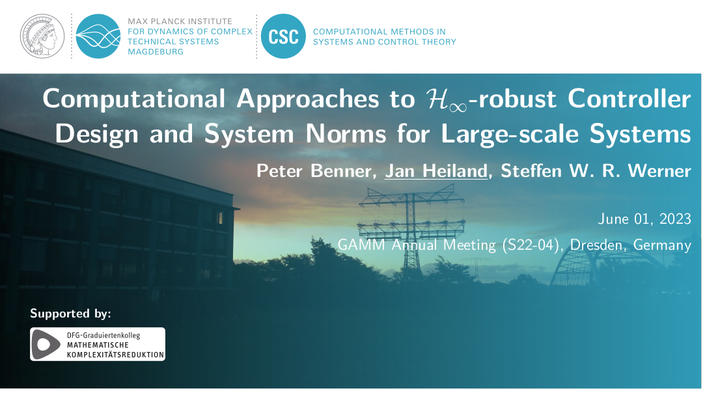# Computational Approaches to H-Infinity-robust Controller Design and System Norms for Large-scale SystemsDate
Jun 1, 2023 18:31 — 18:31
Event
GAMM 2023 annual meeting
Location
TU Dresden
Dresden

The computation of controller gains for the (linearization based) $H_\infty$ robust control of linear (and nonlinear) dynamical systems amounts to the solution of indefinite Riccati equations. For large-scale systems, a direct approach is infeasible because of both computational efforts and memory requirements.

In this talk we review existing iterative approaches and then discuss our recent algorithm1 that merges the ideas of the Riccati iteration2 (that approaches solutions of the indefinite equations through a sequence of definite Riccati equations) and low-rank ADI iterations3 (as it has become a standard tool for solving large-scale Riccati equations numerically). For that we provide necessary and sufficient conditions for convergence and thorough numerical studies concerning run times and memory consumption.

In order to estimate the performance and robustness of controllers, one may resort to system norms which themselves pose a significant computational challenge. In the second part of this talk, we will illustrate suitable reformulations of the relevant system norms so that their estimation can be achieved by high-performant routines from numerical linear algebra4. In view of robust control, we will in particular focus on estimates that also include system uncertainties and model reduction errors.

Finally, we will bring together the computation of the controller gains and the norm estimates to robustly stabilize incompressible flows in two different setups of the well-known cylinder wake.

1. Benner, P.; Heiland, J. & Werner, S. W. R. A low-rank solution method for Riccati equations with indefinite quadratic terms, Numer. Algorithms, 2022 ↩︎

2. Benner, P.; Heiland, J. & Werner, S. W. R.: Robust output-feedback stabilization for incompressible flows using low-dimensional $H_\infty$-controllers. Comput. Optim. Appl., 2022, 82, 225-249 ↩︎

3. Lanzon, A.; Feng, Y. & Anderson, B.: An iterative algorithm to solve Algebraic Riccati Equations with an indefinite quadratic term, 2007 European Control Conference (ECC), 2007. ↩︎

4. Benner, P.; Bujanović, Z.; Kürschner, P. & Saak, J.: RADI: A low-rank ADI-type algorithm for large scale algebraic Riccati equations. Numer. Math., 2018. ↩︎###### Team leader and Jun.-Prof. in Applied Mathematics

My research interests include control systems, differential-algebraic equations, and flow problems.